Problem: Decide whether the following integral converges: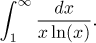Solution: In this integral there are two problems, namely each endpoint. Infinity is obvious, and x = 1 leads to zero in the denominator. Thus we have to split the integral, for instance we can write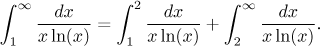We will now look at convergence of the two integrals, starting with the latter. Since this looks like a very simple integral, we will try to evaluate it: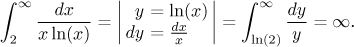(We already know that the last integral is divergent). This means that there is no need to investigate the second part (the integral from 1 to 2), the whole given integral is divergent.

Notes:
1. Note that the convergence of the integral we evaluated above cannot be decided by our tests. First, try the comparison. For x > e we surely have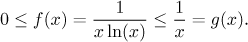Then also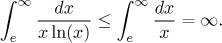and the Comparison test is inconclusive.

We could try to compare the given function to some powers that have convergent integrals to infinity, but this is not possible. This should become clear when we attempt limit comparison. The test function g(x) = 1/x is too large: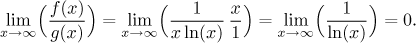If we try a higher power in the denominator, the function will be too small. Precisely, for any p > 1, the choice g(x) = 1/xp leads to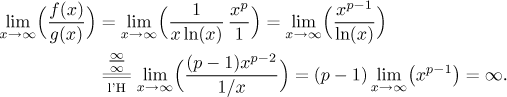Thus powers do not help as test functions in the Limit Comparison test.

Now it is useful to recall the scale of powers at infinity. While 1/x gives a divergent integral, all higher powers give a convergent integral. The given function is squeezed right between these two alternatives. Precisely, the above limit calculation shows that for any given p > 1 we have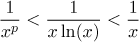for large values of x. This explains why our tests could not work, the given function manages to fall into the gap between convergent and divergent test integrals. We could think of it as a refinement of our test scale. In fact, one can show by evaluating as above that the integral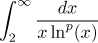converges exactly if p > 1.

2. Although it was not necessary to check on the integral from 1 to 2 to solve our problem, it is nevertheless a good exercise. Since we can easily integrate, we directly evaluate: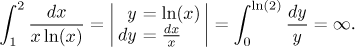So this integral is also divergent. However, logarithm behaves quite differently around 1 as compared to infinity. For instance, while around infinity, logarithm cannot be compared to any power (as we saw above), for values of x close to 1 we have ln(x) ∼ (x − 1); this follows for instance from the theory of Taylor series. Since logarithm is the only source of trouble around x = 1, we can ignore the x in the denominator and try the following choice of test function: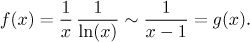Justification now succeeds: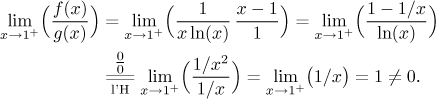Since the test integral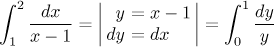diverges, by the Limit Comparison test, also the integral of f from 1 to 2 diverges.